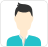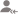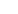true

Find the best tutors and institutes for Class I-V Tuition

Find Best Class I-V Tuition

No matching category found.

No matching Locality found.

Outside India?

Search for topicsPost a Lesson

All

All

Lessons

Discussion

Answered on 06/08/2019 Tuition/Class I-V Tuition Tuition/Class I-V Tuition/MathematicsShashi Bhushan Mishra

Ambitious,enthusiastic and excellent classroom practitioner.Senior Physics and Chemistry Faculty.

Dislike Bookmark

Answered on 12/08/2019 Tuition/Class I-V Tuition Tuition/Class I-V Tuition/MathematicsHarsh Goyal

2000 Rs per month
Dislike Bookmark

Lesson Posted on 17/03/2017 Tuition/Class I-V Tuition Tuition/Class I-V Tuition/MathematicsAmrtha Shashidhar

I am an experienced, qualified teacher and tutor with over 1 year of experience in teaching from Grade...

What are the strategies to calculate mental math addition? The tricky strategies to calculate addition mentally in your head is an important part in mathematics. Year-by-year practicing will help us to learn how to use mental math tricks or techniques in an appropriate way. Tips: Practice whenever... read more

What are the strategies to calculate mental math addition?

The tricky strategies to calculate addition mentally in your head is an important part in mathematics.  Year-by-year practicing will help us to learn how to use mental math tricks or techniques in an appropriate way.

Tips: Practice whenever you get free time in school, at work, etc.,

There are some skills, which are used to solve the questions of addition easily and conveniently. Use mental math strategies to calculate addition to sharpen your mental maths skills.

Here are some examples in which mental math addition skills are used

Problems to calculate mental math tricks for addition:

 1. Add 4 + 8 Solution:    4 + 8 = 8 + 4 4 + 8 = 8 + I, I, I, I          = 8, 9, 10, 11, 12 Therefore, 4 + 8 = 8 + 4 = 12 Instead of adding 8 to 4, 4 is added to 8 as 4 + 8 = 8 + 4. While calculating mentally, we go to count four numbers ahead.
 2. Add 5 + 17 Solution: We know that, 5 + 17 = 17 + 5 So, 17 + (I, I, I, I, I) = 17, 18, 19, 20, 21, 22 Therefore, 5 + 17 = 17 + 5 = 22 Instead of adding 17 to 5, 5 is added to 17 as 5 + 17 = 17 + 5. While calculating mentally, we go to count five numbers ahead.
 3. Add 8 + 5 + 3 Solution: 8 + 5 + 3 = 8 + 3 + 5 = 11 + 5 = 16 Therefore, 8 + 5 + 3 = 8 + 3 + 5 = 16 Instead of adding 8 + 5 + 3 we arrange the numbers as 8 + 3 + 5 then, 8 + 3 = 11 and 11 + 5 = 16
 4. Add 70 + 40 Solution: 70 + 40 = 110 Only the digits at ten’s place are added, if the numbers are the multiples of 10. Zero is placed at one’s place in the addition result.
 5. Add 60 + 35 Solution: 60 + 35 = 60 + 30 + 05 = 90 + 05 = 95 Therefore, 60 + 35 = 95 Numbers are split in tens and ones. Sum of tens and sum of ones are added.
 6. Add 67 + 43 Solution: 67 + 43 = (60 + 7) + (40 + 3) = 60 + 40 + 7 + 3 = 100 + 10 = 110 Numbers are split in tens and ones and then do addition. or,                  67                   43                  10 (7 + 3)                  100 (60 + 40)                 110 Sum of ones and sum of tens both are added.

Solution:

39 + 53

= 39 + 1 + 53 – 1

= 40 + 52

= 92

The above tricky strategies will help us to calculate mental math addition to sharpen the math skills to perform easily.

Dislike Bookmark

Looking for Class I-V Tuition

Find best Class I-V Tuition in your locality on UrbanPro.

FIND NOW

Lesson Posted on 17/03/2017 Tuition/Class I-V Tuition Tuition/Class I-V Tuition/MathematicsAmrtha Shashidhar

I am an experienced, qualified teacher and tutor with over 1 year of experience in teaching from Grade...

What are the strategies to calculate mental math subtraction?The tricky strategies to calculate subtraction mentally in your head is an important part in mathematics. Year-by-year practicing will help us to learn how to use mental math tricks or techniques in an appropriate way. Tips: practice whenever... read more

What are the strategies to calculate mental math subtraction?

The tricky strategies to calculate subtraction mentally in your head is an important part in mathematics.  Year-by-year practicing will help us to learn how to use mental math tricks or techniques in an appropriate way. Tips: practice whenever you get free time in school, at work, etc.,

There are some skills, which are used to solve the questions of subtraction easily and conveniently. Use mental math strategies to calculate subtraction to sharpen your mental maths skills.

Here are some easy examples in which mental subtraction skills are used.

Problems to calculate mental math tricks for subtraction:

1. Subtract 27 from 73.

Solution:

73 – 27

= 73 + 99 – 27 – 99

= 73 + 99 – 27 – 100 + 1

= 73 + (99 – 27) - 100 + 1

= 73 + 72 - 100 + 1

= (73 + 72) – 100 + 1

= 145 - 100 + 1

= 45 + 1

= 46

Therefore, 73 – 27 = 46

For the solution we deduct 27 from the highest two-digit number.

 99           -   27               72           +  73             145          -  100               45          +     1                46 Now 73 is added to 72 (72 + 73 = 145) Again from the sum 100 is deducted and then 1 is added. 72 + 73 → 145 – 100 → 45 + 1 = 46 This 46 is the subtracted result of 73 – 27

2. Subtract 93 – 67

Solution:

93 + (99 – 67) – 100 + 1

= 93 + 32 - 100 + 1

= (93 + 32) – 100 + 1

= 125 – 100 + 1

= 25 + 1

= 26

Therefore, 93 – 67 = 26

3. By backward counting subtract 5 from 19.

Solution:

19

-  5
14

19 – 5

= 19 –  I,     I,      I,      I,      I

18,    17,   16,    15,    14

Therefore, 19 – 5 = 14

4. Find the difference of 53 – 29

Solution:

53           59     →    30      →

-  29          -29             -  6
30               24

Hence,          53

–     29
24

The above tricky strategies will help us to calculate mental subtraction to sharpen the math skills to perform easily.

Dislike Bookmark

Lesson Posted on 21/02/2017 CBSE/Class 10/Mathematics Tuition/Class I-V Tuition Tuition/Class I-V Tuition/Mathematics +2Amrtha Shashidhar

I am an experienced, qualified teacher and tutor with over 1 year of experience in teaching from Grade...

Exponential Form = 100 = 102, where 2 is the power of 10. Laws of exponents: a-m = 1/ am a m * an = am*n am * an = am*n (am)n = am*n am / bm = (a/b)m a0= 1 (a / b)-m = (b/a)m (-1)odd = -1 (-1)even = 1 ( am / an ) = am-n read more

Exponential Form = 100 = 102,

where 2 is the power of 10.

Laws of exponents:

1. a-m = 1/ am
2. a m * a= am*n
3. am * a= am*n
4. (am)= am*n
5. am / b= (a/b)
6. a0= 1
7. (a / b)-m = (b/a)
8. (-1)odd = -1
9. (-1)even = 1
10. ( am / an ) = am-n

Dislike Bookmark

Answered on 31/12/2016 Tuition/Class I-V Tuition Tuition/Class I-V Tuition/MathematicsX%of y= x/100*y.
Dislike Bookmark

Answered on 27/10/2016 Tuition/Class I-V Tuition Tuition/Class I-V Tuition/Mathematics360cm
Dislike Bookmark

Lesson Posted on 12/09/2016 CBSE/Class 10/Mathematics CBSE/Class 12/Science/Mathematics Tuition/Class IX-X Tuition +5 Tuition/Class I-V Tuition Tuition/Class I-V Tuition/Mathematics Tuition/Class VI-VIII Tuition Tuition/Class VI-VIII Tuition/Mathematics Tuition/Class XI-XII Tuition (PUC) lessFirst thing i would like to say that once i take ur responsibility i will work with u till ur fulfilment...

Its understanding of urs about the maths that matters a lot. There are some learning tips which helps you a lot. 1. Be relaxed and dont take too much tension. 2. Try to learn concept first and then think over it . 3. Write formulaes of maths in a A4 sheet and paste in your bed room and recall all... read more

Its understanding of urs about the maths that matters a lot.

There are some learning tips which helps you a lot.

1. Be relaxed and dont take too much tension.

2. Try to learn concept first and then think over it .

3. Write formulaes of maths in a A4 sheet and paste  in your bed room and recall all while taking rest.

4. Do as much practice u can .

So  these r some of the basic tips which will help you to stand out the tough.

Dislike Bookmark

Answered on 30/08/2016 CBSE/Class 10/Mathematics Tuition/Class I-V Tuition Tuition/Class I-V Tuition/Mathematics +1Aparna G.

MBA Finance trainer

Join Urbanpro because it is very reasonable in comparison to others or u can join some bureaus who are providing tuition services. U can find those bureaus on Facebook page to search over there as HOME TUTORS title.
Dislike Bookmark

Looking for Class I-V Tuition

Find best Class I-V Tuition in your locality on UrbanPro.

FIND NOW

Answered on 01/01/2018 Tuition/Class I-V Tuition Tuition/Class I-V Tuition/MathematicsHighly Qualified Tutor

12 + 11 = 23
Dislike Bookmark

UrbanPro.com helps you to connect with the best Class I-V Tuition in India. Post Your Requirement today and get connected.225,708 Followers

## Top Contributors

Connect with Expert Tutors & Institutes for Mathematics

## Class I-V Tuition in:

x

X

### Looking for Class I-V Tuition Classes?

Find best tutors for Class I-V Tuition Classes by posting a requirement.

• Post a learning requirement
• Get customized responses
• Compare and select the best### Looking for Class I-V Tuition Classes?

Find best Class I-V Tuition Classes in your locality on UrbanProUrbanPro.com is India's largest network of most trusted tutors and institutes. Over 55 lakh students rely on UrbanPro.com, to fulfill their learning requirements across 1,000+ categories. Using UrbanPro.com, parents, and students can compare multiple Tutors and Institutes and choose the one that best suits their requirements. More than 7.5 lakh verified Tutors and Institutes are helping millions of students every day and growing their tutoring business on UrbanPro.com. Whether you are looking for a tutor to learn mathematics, a German language trainer to brush up your German language skills or an institute to upgrade your IT skills, we have got the best selection of Tutors and Training Institutes for you. Read more